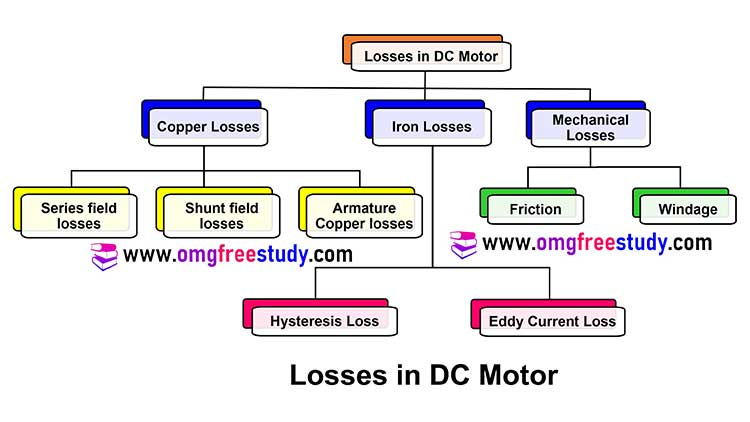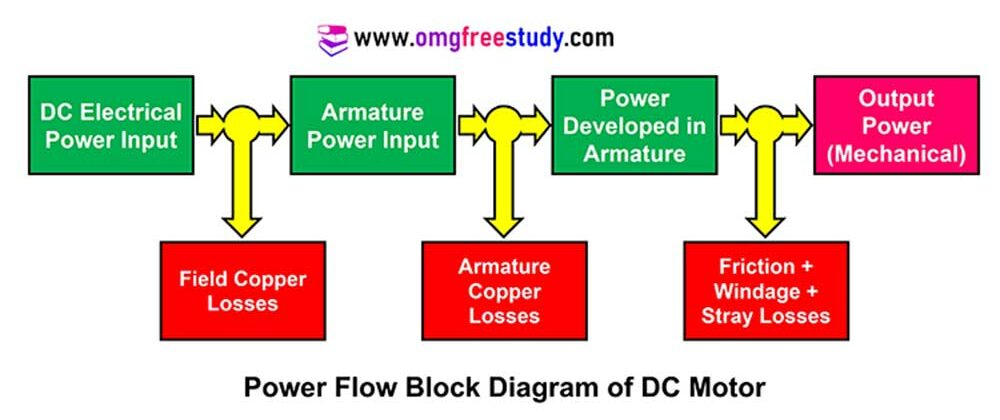# Losses in DC Machines | Power Stage Flow Diagram

The losses in DC machines are divided into five basic categories. These various losses are copper loss or I2R loss, Iron loss or core loss, mechanical loss, Brush Loss and stray loss. All this losses are explained in detail below.

## Losses in DC Machines

Various losses in dc machines are shown in tree diagram which is given below.Que. List the various losses on a DC Motor.

The various losses on a DC Motor are,

1) Winding losses (Copper losses)

• Armature winding losses
• Shunt field losses
• Series field losses

2) Core losses (iron losses)

3) Mechanical loss

• Brush contact losses
• Windage and frictional

### Copper or Electrical Losses

• The copper losses occur due to the resistance offered by the winding. Generally winding is made up of copper. Hence, these losses are also referred as winding losses.
• This loss is also called as variable loss, because this loss mainly depend upon current and current can change with load on machine.
• Mathematically, copper losses can be given as I2 R.
• Armature copper losses, Ia2 Ra, are around 30% of the total full-load losses.
• Similarly shunt copper losses are Ish2 Rsh and series copper losses are Ise2 Rse.

### Core Losses or Iron Losses

• These losses are due to hysteresis or the eddy current losses.
• Such losses are also called as magnetic losses, and also this loss is called as constant loss.
• When the dc machine is operated at constant flux density and at constant speed, these losses do not very much.

### Brush Loss

• Power losses also occurs at point of constant between copper commutator and the carbon brush.
• This loss is also very small as compared with all other losses in machine.

### Mechanical Loss

• Losses occurring due to mechanical affects, like friction etc. are called as mechanical losses.
• Mechanical losses include bearing friction loss and windage loss.
• These losses are very small in magnitude as compared to copper loss and iron loss.

### Stray Loss

all losses which is discus above are classified under stray loss. These loss also called as miscellaneous loss because they depend on factor like distortion in flux, current variation due to armature reaction etc.

Generally these losses are consider to be in very small value is 1% nearly of full load power output.

## Power Stage Flow Diagram

Que. Draw power stages of DC motor Diagram.Watch MCQ Question Lecture on DC Motor and DC Gen. on YouTube by clicking here.

Scroll to Top
Scroll to Top